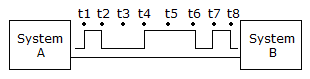# Digital Electronics - Digital Concepts

### Exercise :: Digital Concepts - Filling the Blanks

1.

Digital systems have ________.

 A. one state B. two states C. three states D. four states

Answer: Option B

Explanation:

No answer description available for this question. Let us discuss.

2.

The systems shown in the given figure transfer data ________.A. serially B. sequentially C. in parallel D. both serially and sequentially

Answer: Option D

Explanation:

No answer description available for this question. Let us discuss.

3.

Digital systems are called ________.

 A. binary systems B. logic systems C. numbering systems D. ADC systems

Answer: Option B

Explanation:

No answer description available for this question. Let us discuss.

4.

1/4 as a binary number would be ________.

 A. 0.01 B. 0.11 C. 0.1 D. 0

Answer: Option A

Explanation:

No answer description available for this question. Let us discuss.

5.

The invalid range for an input to TTL logic is from ________.

 A. 0 to 0.8 V B. 1.2 to 1.6 V C. 0.8 to 2.0 V D. 2.0 to 5.0 V

Answer: Option C

Explanation:

No answer description available for this question. Let us discuss.

#### Current Affairs 2021

Interview Questions and Answers